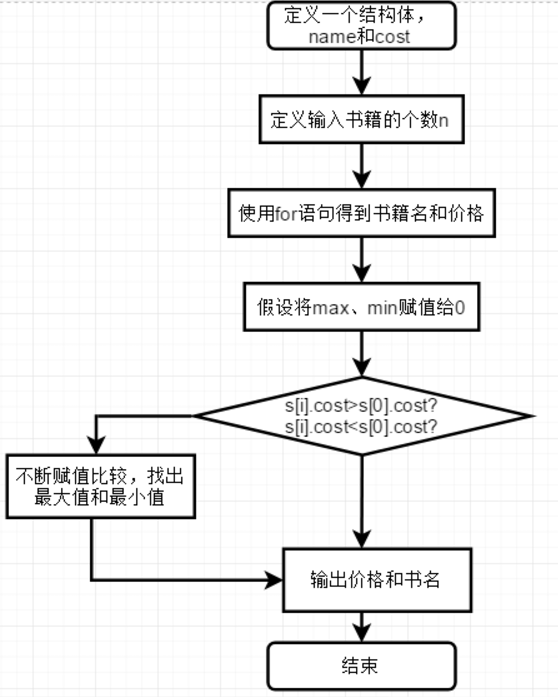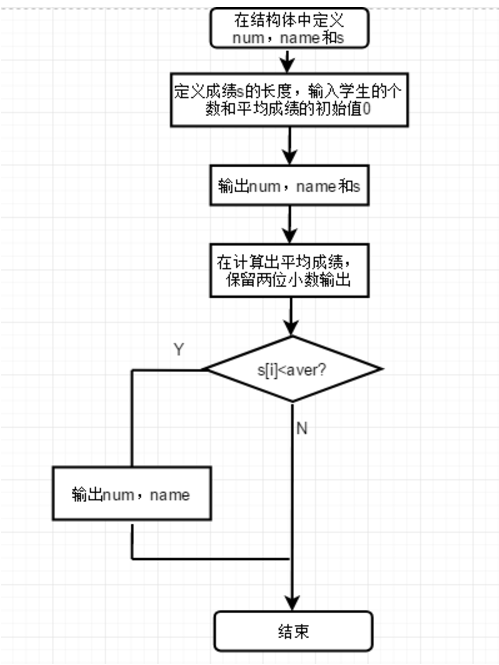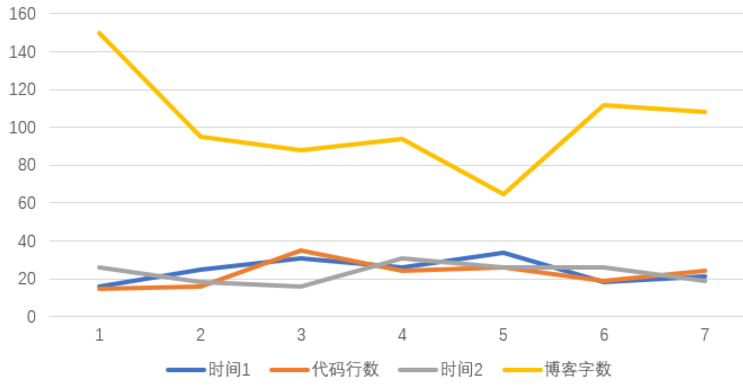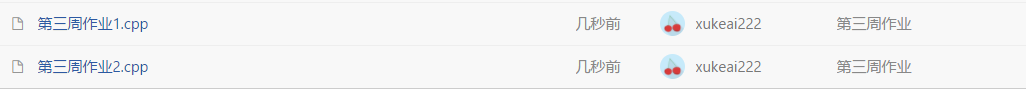struct sk{int a; char str)}p; p->str++ 中的++ 加向str

1 设计思路
（1）主要描述题目算法#include <stdio.h>
#include<stdio.h>
struct book
{
char name;
double price;
}b;
int main()
{
int n,i,min=0,max=0;
scanf("%d",&n);
for(i=0;i<n;i++)
{
scanf("\n");
gets(b[i].name);
scanf("%lf",&b[i].price);
if(b[i].price>b[max].price)
{

max=i;
}
if(b[i].price<b[min].price)
{

min=i;
}
}
printf("%.2lf, %s\n",b[max].price,b[max].name);
printf("%.2lf, %s\n",b[min].price,b[min].name);
return 0;
}  

1 设计思路
(1)主要描述题目算法代码

#include<stdio.h>
struct score
{
char num;
char name;
double s;
};
int main()
{
struct score s;
int i,n;
double sum=0,average;
scanf("%d",&n);
for(i=0;i<n;i++)
{
scanf("%s%s%lf",&s[i].num,&s[i].name,&s[i].s);
}
for(i=0;i<n;i++)
{
sum=sum+s[i].s;
}
average=sum/n;
printf("%.2f\n",average);
for(i=0;i<n;i++)
{
if(s[i].s<average)
{
printf("%s %s\n",s[i].name,s[i].num);

}
}
return 0;
}

1、指针与结构作业中题目所使用的知识点。
1.结构体的正确使用和输出定义的变量。git截图git 链接

https://coding.net/u/xukeai222/p/XUJINGQI/git/tree/master/?public=true